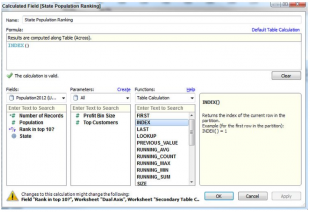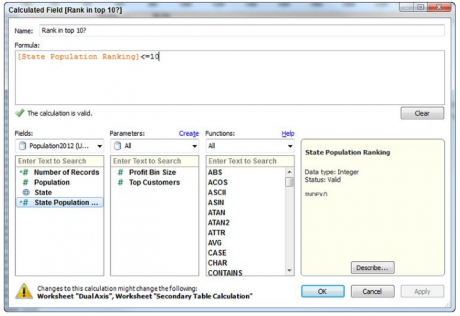Home / Tableau

# How to Use Table Calculation Functions in Tableau

Rating: 4.0Views: 3241
by Saikumar

Using Table Calculation Functions

Use table calculation functions to customize table calculations. Table Calculations are computations that are applied to the values in the entire table and are often dependent on the table structure itself.
The index function is a kind of table calculation function that counts the position of a row or column in a set. A calculated value called state population ranking was created using this function. Below shows the calculated value using the index function.

Accelerate your career with Best Tableau Training and become expertise in Tableau.

Creating the Boolean calculated value compares the result of the index to a top 10nranking value. The resulting calculated value is placed on the color shelf for the bar chart to encode the top 10 states with a different color. Below diagram shows the Boolean formula being created.Creating a population rank diagramCreating the Boolean calculation diagram

The Boolean formula in the lower most diagram compares the state population ranking with the number 10 to derive a true-false condition for the top 10 ranked states by population. The resulting Calculated Value is then added to the color button on the Marks card. The resulting color encoding is seen in that diagram only.

Related Page: How To Install The Command Line Tools In Tableau?

Using Table Calculations in combination with Calculated Values that employ Table Calculation Functions helps you add more meaning and context for analysis. There is really no limit to the creative ways you can use Calculated Values and Table Calculations to enhance the information.

### Related Pages:

Tableau Server Tutorial

Tableau Interview Questions

Tableau Sample Resumes

List Of Tableau Courses: